When a company grows larger, the needs for travel will grow, which brings an increase in travel costs. In order to effectively track these travel costs, you need to have a travel policy that works for your company.

Companies can provide travel budget templates that allow company travelers to fill in travel data in a default format, which can greatly improve the efficiency of managing company travel budgets.

It is important to manage your business travel expenses for the growth of your company. Here are some things you need to consider when making your business travel budget.

• Company’s travel policy
• Number of possible company trips or destinations during the year
• Cost of travel transportation
• Need to consider seasonal or external factors
• Establish a clear business travel policy

This business Trip budget template is officially provided by Microsoft Excel and you can create the template directly in the Excel application or download it from the official website.

You can use this business travel budget template to track every expense item of your business trip.

This template contains only contains worksheet：Business trip budget.

Where,

• Business trip budget: This worksheet contains the total budget amount and a breakdown of all expense items for the trip.

Let’s see how to use this Business trip budget template:

Step1: Open Microsoft Excel Spreadsheet, click on the File menu, and then click on the New submenu

Step2: In the New dialog box, find the template search box, type in the keyword ” Business trip budget ” and press Enter, you will see the Business trip budget template.

Step4: You need to enter Target trip budget firstly in cell C3 in the Business trip budget worksheet.

Step5: You need to enter Trip details in Data table in Business trip budget worksheet.

Step6: Total trip cost and balance are automatically calculated by one Excel Subtotal formula.

``=SUBTOTAL(109,[Amount])``

If you are looking for Business trip budget  template, check out Microsoft office site by clicking here.

## Wedding Expense Budget Template

One of the trickiest things about weddings is figuring out the breakdown of your wedding budget. In order to figure out the exact breakdown of your wedding costs, such as the wedding budget percentages for catering, costumes, flowers, venue fees and music, you can create a detailed wedding budget spreadsheet that will help you keep track of the budget breakdown for all the cost categories for your wedding event.

Before you can create a wedding budget, you first need to determine the total budget for your wedding event. After that, you need to know how to allocate the budget. You can refer to the wedding budget breakdown given in the wedding budget template, which can greatly simplify the process for you.

This article will provide a free EXCEL wedding budget template that you can use to track your wedding event based on your estimated and actual costs. This wedding budget template also shows the percentage of the total budget each category takes up with a rising sun chart and highlights the expenses for each category with arrows to show items that are over or under budget.

## Wedding expense budget

This wedding expense budget is officially provided by Microsoft Excel and you can create the template directly in the Excel application or download it through the official website.

You can use this Wedding expense budget template to track the breakdown of the different expense categories for your wedding.

This template contains 3 sheets：Start, Wedding Budget, Expenses.

Where，

• Start: This sheet contains usage instructions of the Wedding Budget Template.
• Wedding Budget: This sheet summarizes the estimated and actual amounts spent on different items for wedding events.
• Expenses: This sheet contains a breakdown of the costs of the different items in the wedding event.

Let’s see how to use this wedding budget template:

Step1: Open Microsoft Excel Spreadsheet, click on the File menu, and then click on the New submenu

Step2: In the New dialog box, find the template search box, type in the keyword ” Wedding expense budget ” and press Enter, you will see the Wedding expense budget template.

Step3: Click the Create button to download the selected Wedding expense budget   template to create a new Wedding expense budget spreadsheet.

Step4: You can see an introduction of the usage of the wedding budget template in the start worksheet.

Step5: You need to enter Estimated and Actual costs for each category in respective tables in Expenses worksheets.Over or under amount is automatically calculated.

Note: The Total value in each category table is automatically calculated using the SUBTOTAL formula.

Step6: Once you enter the expense details for the different categories in the expense worksheet, you can see the estimated total and actual total expenses for the different categories in the wedding budget worksheet, and a Rising Sun chart lets you see at a glance how much of the total budget each category is taking up.

If you have a need for a custom budget template, you can leave us a comment.

If you are looking for Wedding budget template, check out Microsoft office site by clicking here.

## Monthly College Expense Budget

For college students, a college budget is a key element to help them manage their money and make sure they don’t overspend. When you want to create a college budget, you can start by figuring out all the expense and income items, and then you can create your college budget through Microsoft Excel application or Google Sheets online spreadsheet.

Most college students will have a variety of different income and expense items. Income items may come from family members, grants, loans, or money you earn from your job. Expense items may include monthly necessities, tuition, rent, internet, car repairs, parking, credit card payments, books, or medical expenses.

Expense items may include variable costs, such as dining out, entertainment, clothing, cabs, etc.

In order for you to juggle savings, grants, scholarships, student loans or other income to cover your tuition or other variable costs, then you can make a college budget spreadsheet to track all of your income and expenses.

## Monthly college expense budget

This Monthly college expense budget is officially provided by Microsoft Excel, you can create the template directly in the Excel application or download the template from the official website.

You can use this monthly college expense budget template to track your monthly expenses and income while you are in college.

This template contains one sheet：Income and expenses.

Where,

• Income and expenses: This sheet contain a breakdown of monthly income items and expense items, as well as a breakdown of semester expenses.

Let’s see how to use this college budget template:

Step1: Open Microsoft Excel Spreadsheet, click on the File menu, and then click on the New submenu

Step2: In the New dialog box, find the template search box, type in the keyword ” Monthly college expense budget ” and press Enter, you will see the Monthly college expense budget template.

Step3: Click the Create button to download the selected Monthly college expense budget   template to create a new Monthly college expense budget spreadsheet.

Step4: You need to enter the appropriate data in the Monthly Income, Monthly Expenses, and Semester Expenses tables at the bottom of the Income and Expenses worksheet, such as a breakdown of different income items, a breakdown of different expense categories, and a breakdown of different semester expense items.

Note: The total data for each table is automatically calculated using the SUBTOTAL formula.

Step5: Once you have entered the corresponding income and expense data, the template will display the different income or expense categories in the form of a bar chart.

If you have a need for a custom budget template, you can send us a message.

If you are looking for Family budget template, check out Microsoft office site by clicking here.

## Event Budget Simple Template

A proper event planning is one of the most important factors for a successful event. Before you can create an event plan, you need to set goals and the budget needed for the event. The budget spreadsheet is a detailed projection of the financial situation at the event.

You may end up spending more than you expected. Therefore, you need to follow the budget items in the budget form to help control your expenses and income and to measure the success of your event.

Event budget planning is time consuming to develop and manage, and it is worth spending that time. You need to predict as accurately as possible whether the event will produce a profit, loss or break-even result. To achieve this, you need to list all the expenses and incomes that the event is likely to generate, and you need to track and review the budget regularly to ensure that your funds are allocated to the correct resources.

This article will introduce a free event budget template that will help you track estimated and actual costs as well as estimated and actual incomes for your event. The template also shows profits and losses in graphical form.

## Event budget simple

This Event budget simple template is officially provided by Microsoft Excel, you can create the template directly in the Excel application or you can download the template through the official website.

You can use this Event budget simple template to track the estimated and actual costs of your events as well as the estimated and actual incomes.

This template contains 3 sheets：Expenses, Income, Profit & loss summary.

Where,

• Expenses: This sheet contains a breakdown of expenses for the different cost categories in the event.
• Income: This sheet contains a breakdown of incomes for the different cost categories in the event.
• Profit & loss summary: This sheet contains summary statistics of expenses for the different expense categories of the event, as well as summary statistics of incomes for the different income categories, and also contains a profit and loss chart for the event.

Let’s see how to use this event budget template.

Step1: Open Microsoft Excel Spreadsheet, click on the File menu, and then click on the New submenu

Step2: In the New dialog box, find the template search box, type in the keyword ” Event budget simple ” and press Enter, you will see the Event budget simple template.

Step3: Click the Create button to download the selected Event budget simple template to create a new Event budget simple spreadsheet.

Step4: You need to enter Income details in Expenses worksheets.

All Total rows are automatically calculated via Excel SUBTOTAL function.  For example:

``=SUBTOTAL(109,[Estimated])``

Step5: You need to enter Income details in Income worksheets.

Total Rows for each income subcategory are automatically calculated by the SUBTOTAL function with the following formula.:

``=SUBTOTAL(109,[Estimated])``

The projected and actual total income in the top Total income table is calculated automatically by using the SUM function.

``=SUM(Admissions[[#Totals],[Actual income]],AdsInProgram[[#Totals],[Actual income]],ExhibitorsAndVendors[[#Totals],[Actual income]],SaleOfItems[[#Totals],[Actual income]])``

Step6: Once you have entered the corresponding event expense and income details in the Expenses and Income worksheets, you can see that the Income Statistics and Expense Statistics tables in the Profit & loss summary worksheet are automatically updated.

The Profit and Loss Table and Profit and Loss Bar Chart are also updated automatically.

If you have a need for a custom budget template, you can send us a message.

If you are looking for Family budget template, check out Microsoft office site by clicking here.

## Free Family Budget Template (Step-by-step Guide)

This article will show you some free printable family budget templates for google sheet and Microsoft Excel spreadsheet applications and will also explain some of the features or important functions of these templates.

This will make it easy for you to make modifications when using these templates so that you can customize the family budget template to meet your specific requirements.

## What Is A Family Budget?

A budget is simply a plan for your money – everything that comes in (income) and goes out (expenses). A family budget is a plan you make for your entire family. The best family budgets include everyone in the family.

A family budget is a budget plan used for the family’s monthly or annual income and expenses to determine the balance of the family’s savings or debts.

A family budget is a plan you create based on your family’s income and expenses. This is a plan for all of your spending amounts, which also includes money for recreational activities or personal entertainment spending, so creating a solid budget plan can help you achieve your family’s financial goals.

Family budgeting can include a comprehensive list of household income or expenses, and you can focus on several categories. You can write a budget plan manually or design a reliable, printable family budget spreadsheet by using Google Sheets or Microsoft Excel Spreadsheet.

## Why Is A Family Budget Important?

A budget can be of great benefit to every family, not just to those currently in financial distress. A family budget plan encourages you to spend on reasonable spending items.

It encourages you to live within your means and put your money to work in the best way possible. Using a budget plan is one way to achieve your financial goals. It can help you.

• Track your income and expenses, so you know exactly how much your family is saving or spending each month
• Eliminate unnecessary spending; you can eliminate unnecessary spending by following the expense items in your household budget spreadsheet
• Helps you plan ahead and use available funds for emergency aggressive or savings goals to prepare for future retirement
• Relieving financial stress and proper planning can help you have a healthy family financial situation

If you only want to manage your family’s finances with a calculator and a notebook, you may be thinking a little simplistically. Whether you live alone or have a large family, there are many expense items, so you need to create a detailed family financial planning that gives you a clearer overview of your current spending, helps you guide your savings, and identifies where you should spend your money wisely.

With the Family Expense tracker Spreadsheet, you can have a good control about your family’s financial situation.

## What Is A Family Budget Template?

A Family budget template is a predefined solution that helps you categorize and analyze your household finances for a specific period of time, such as a month, quarter or year.

Each template contains different features, but it is usually designed in an easy-to-use format to help you manage your family’s money.

In short, a family budget template should be easy to use. You can imagine all of your monthly bills and cash received. When you break down your monthly expenses, these should match your income enough so that you can pay bills, save money and achieve other financial goals.

## Free Family Budget Templates

Below we will share with you some free printable, customizable family budget templates. These templates can be opened and used in Microsoft Excel spreadsheets or Google Sheets. You can download them for free and print them out to use.

### 1. Free Family Budget by Microsoft

This template is an official household budget template provided by Microsoft Excel, if you need to use this template in google sheets, then you just need to import the budget template file into google sheets.

You can use this family budget worksheet to track your family’s projected or actual total income and projected total expenses and actual costs.

This template contains 3 worksheets: Cash Flow, Monthly Income, Monthly Expenses; you can use these three worksheets to count your family’s monthly income and income and expenses.

The Cash Flow table contains a Cash Flow chart, and the data in this chart is also automatically grabbed from the Monthly Income and Monthly Expenses worksheets and automatically updated into the chart.

Let’s take a look at how to use this family budget template.

STEP 1: First, you need to enter the family name in cell B3 of the Cash Flow worksheet, and enter the year and month in cell B5.

STEP 2: Go to the Monthly Income Worksheet and enter the expected and actual income values for all income items for the entire family.

This table also automatically sums all projected and actual revenue items, using the following formula：

```=SUBTOTAL(109,[Projected])

=SUBTOTAL(109,[Actual])```

STEP 3: You need to go to the Monthly Expenses worksheet and enter the expense amounts for different expense categories.

This worksheet also contains an automatic summation of all projected and actual spend items. The formula used is as follows.

```=SUBTOTAL(109,[Projected])

=SUBTOTAL(109,[Actual])```

STEP 4: When you enter the values of income and expense items in the Monthly Income and Monthly Expenses worksheets, you will see that the Cash Flow chart in the Cash Flow worksheet will also be updated automatically, and the values of Total Income and Total Expenses will also be updated automatically.

You may be wondering how the total projected income, total actual income, total projected expense, and total actual expense values in the Cash Flow worksheet are extracted from the other two worksheets? All the data is extracted using a formula similar to the following:

```=Income[[#Totals],[Projected]]

=Expenses[[#Totals],[Projected]]```

Where income and expenses are the two defined name ranges:

income = ‘monthly income’\$ B\$6:\$E\$8

Expenses = ’Monthly Expenses’!\$ B\$6:\$E\$25.

If you have other needs for a customized budget template, you can leave us a comment.

If you are looking for Family budget template, check out Microsoft office site by clicking here.

### 2. Free Monthly Family Budget by Microsoft

This template is an official monthly family budget template provided by Microsoft Excel, if you need to use this template in google sheets, then you just need to import the budget template file into google sheets.

The template has only one worksheet which contains all the monthly family income and expense items that need to be tracked and contains various statistical items such as projected monthly income items, actual monthly income items, monthly balance.

The template tracks projected and actual expenses for the entire month through multiple budget categories, including 12 main expense categories: Housing, Transportation, Insurance Food, Children, Legal, Savings/Investments, Loans.  Entertainment, Taxes, Personal Care, Pets, Gifts and Donations.

Let’s see how to use this monthly household budget spreadsheet template.

STEP 1: First you need to enter the projected family income values for the different income items in the Projected Monthly Income Source form.

STEP2: You will need to enter the actual family income values for the different income items in the Actual Monthly Income Source table.

Both tables above will sum up each income item by using the SUM function. The formula used for this is as follows.

`=SUM(H11:H13)`

STEP 3: The most important step in the family budget table is to enter the projected monthly expense item values and the actual monthly expense item values in the different expense category tables.

For example, you can keep track of the expenses related to the Housing expense category in the Housing table.

In addition, the values of each expense sub-category are summed up in each expense category table by the SUBTOTAL formula, the formula is as follows.

`=SUBTOTAL(109,[Projected Cost])`

STEP 4: Once you have entered all the expense item values, you can see the total estimated expense amount, total actual expense amount, and total expense difference information from the Summary table. These statistics will be updated automatically.

If you have other needs for customizing the budget template, please send us a message.

If you are looking for Family budget template, check out it  by clicking here.

### Related Functions

• Excel SUM function
The Excel SUM function will adds all numbers in a range of cells and returns the sum of these values. You can add individual values, cell references or ranges in excel.The syntax of the SUM function is as below:= SUM(number1,[number2],…)…
The Google Sheets SUBTOTAL function returns the subtotal of the numbers in a list or database. The syntax of the SUBTOTAL function is as below:= SUBTOTAL (function_num, ref1, [ref2])….

## How to Subtotal Values for Groups and Only Keep One Subtotal for A Group in Column

Sometimes we may meet the case that subtotal values for different groups and only record a subtotal value for one group in a column, for other cells in this group, keep them blank. Today we will introduce you how can we subtotal values for groups and record subtotal values only in specific cells.

We can get subtotal through Subtotal function or SUM function in simple cases, but per different requirement, we need to apply different functions or combinations. Today we will provide a formula contains three functions IF/COUNTIF/SUMIFS to solve this issue. Through demonstrate a simple instance, we will introduce you the syntax, arguments of these functions, and let you know how the formula works step by step. After reading the article, you can think about if there are some other ways to solve this problem.

## EXAMPLE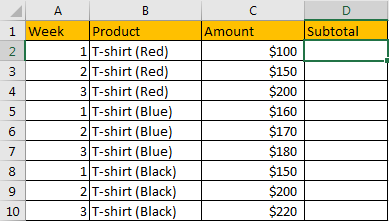Refer to above table, we can see that T-shirts are grouped by colors, and amounts for weeks are separately listed. Our expectation is “get subtotal for each product and record them in proper cell”, see example below: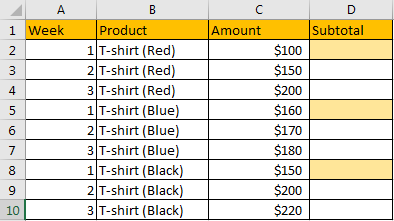We want to 1) save the subtotal values in the cells which background is filled in light orange. 2) For the other cells in this column should keep blank. 3) By the way, we also want to enter only one formula into D2, then though dragging the handle down to fill other cells, subtotal in D5 and D8 should also be calculated properly.

Can we create a formula that can cover above three conditions? Actually, yes.

In this instance, with the help of IF, COUNTIF and SUMIF functions, we can calculate subtotal for each product by only one formula properly.

## FORMULA

Step 1: Select B2:B10, then in Name Box define a new name for this range, for example ‘Product’.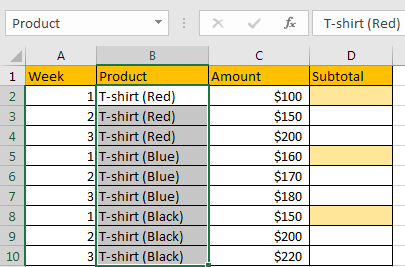Step 2: Select C2:C10, in Name Box define a new name for this range, for example ‘Amount’.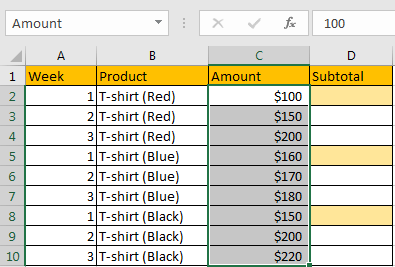Step 3: In D2, enter the formula =IF(COUNTIF(\$B\$2:B2,B2)=1,SUMIF(\$B\$2:\$B\$10,B2,\$C\$2:\$C\$10),””).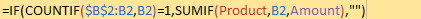NOTE: In step#1 and step#2 we defined “Product” and “Amount” two ranges, when entering the formula, after typing “Amou…”, defined range “Amount” is auto loaded. User defined range can be seen as absolute reference, so when copy formula to other cells, the reference will not be adjusted automatically. If we use range reference B2:B10 in the formula, we need to add “\$” to lock the range.

Step 4: Press Enter after typing the formula. A number is returned.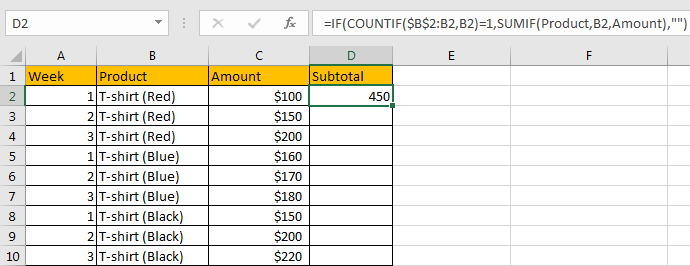We can see in column D2, subtotal \$450 is returned, it is equal to 100+150+200, the total of C2:C4. The formula is correct.

Step 5: Drag the handle down to fill other cells.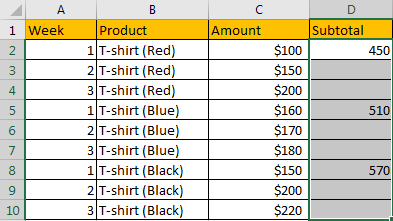Verify that subtotal values are calculated correctly for each product. Besides, the subtotal is only recorded in the first row of each group, others keep blank.

## FUNCTION INTRODUCTION

In this formula we create a formula with IF function which applies COUNTIF and SUMIF functions as its arguments.

IF function will run a logical test first, and based on the result ‘True’ of ‘False’, it chooses to which branch to execute.

For IF function, the syntax is:

=IF (logical_test, [value_if_true], [value_if_false])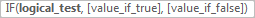———————————————————————————————-

COUNTIF function can used for counting cell number based on different criteria.

For COUNTIF function, the syntax is:

=COUNTIF (range, criteria)

———————————————————————————————-

SUMIFS function can be seen as SUM+IFS, it can handle multiple ‘criteria range’ and ‘criteria’ combinations.

For SUMIF function, the syntax is:

=SUMIF (range, criteria, [sum_range])

For above three functions, they all allow logical operators like “>”,”>”,”<>” and wildcards like “*”,”?”.

## FUNCTION ARGUMENTS EXPLANATION

For If function:

Logical_test: COUNTIF(\$B\$2:B2,B2)=1

value_if_true: SUMIF(\$B\$2:\$B\$10,B2,\$C\$2:\$C\$10)

value_if_false: “” – nothing returns

——————————————————————————————————————–

For COUNTIF function:

Range: \$B\$2:B2

Criteria: B2

For range \$B\$2:B2 is an expanding range. Start cell is B2, and the end cell is not fixed.

Select range and criteria separately in the formula bar, and press F9 to convert cell reference to real values. See screenshot below: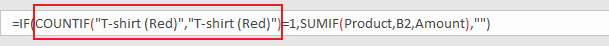——————————————————————————————————————–

For SUMIF function:

Range: \$B\$2:\$B\$10

Criteria: B2

Sum Range: \$C\$2:\$C\$10

Select above arguments, press F9 to convert cell reference to real values. See screenshot below: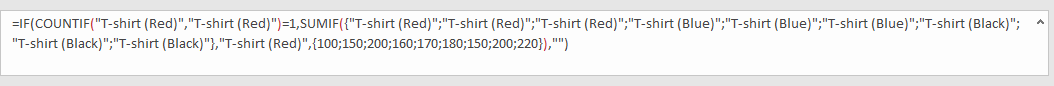## HOW THIS FORMULA WORKS

After explaining each argument in the formula, now we will show you how the formula works with these arguments.

Refer to above steps, the formula is converted to below format in the formula bar.

`=IF(COUNTIF("T-shirt (Red)","T-shirt (Red)")=1,SUMIF({"T-shirt (Red)";"T-shirt (Red)";"T-shirt (Red)";"T-shirt (Blue)";"T-shirt (Blue)";"T-shirt (Blue)";"T-shirt (Black)";"T-shirt (Black)";"T-shirt (Black)"},"T-shirt (Red)",{100;150;200;160;170;180;150;200;220}),"")`

——————————————————————————————————————–

In IF function, the core part is logical test part, its returned value determines which branch we will go to.

Logical_test:

`COUNTIF("T-shirt (Red)","T-shirt (Red)")=1`

For this part, range “T-shirt (Red)” just contains one criteria “T-shirt (Red)”, so COUNTIF(“T-shirt (Red)”,”T-shirt (Red)”)=1, the formula COUNTIF(“T-shirt (Red)”,”T-shirt (Red)”)=1 is ‘True’. So we go to ‘value_if_ture’ and ignore ‘value_if_false’.

value_if_true:

`SUMIF({"T-shirt (Red)";"T-shirt (Red)";"T-shirt (Red)";"T-shirt (Blue)";"T-shirt (Blue)";"T-shirt (Blue)";"T-shirt (Black)";"T-shirt (Black)";"T-shirt (Black)"},"T-shirt (Red)",{100;150;200;160;170;180;150;200;220})`

For this part, we have one pair of criteria range and criteria:

criteria range:

`{"T-shirt (Red)";"T-shirt (Red)";"T-shirt (Red)";"T-shirt (Blue)";"T-shirt (Blue)";"T-shirt (Blue)";"T-shirt (Black)";"T-shirt (Black)}`

criteria:

`"T-shirt (Red)"`

Compare each value in the array with criteria, we get a new array that only contains ‘True’ and ‘False’.

`{True;True;True;False;False;False;False;False}`

Convert True to 1, False to 0.

{1;1;1;0;0;0;0;0}

Now, we have below two arrays in the formula:

Sum Range:

`{100;150;200;160;170;180;150;200;220}`

{1;1;1;0;0;0;0;0}

We list the two arrays in two rows, multiply the two numbers in the same column, and save their products in another row, sum all products together, we can get 450 at last.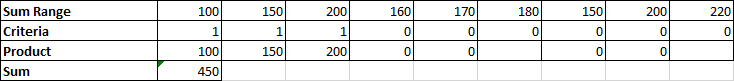## NOTE

We noticed that after typing the formula and dragging the handle down to fill the ‘Subtotal’ column, not all the cells have a sum value, some cells keep blank as we expect. Why the formula returns an empty value after coping it to other cells?

In cell D3, the formula is automatically adjusted to =IF(COUNTIF(\$B\$2:B3,B3)=1,SUMIF(Product,B3,Amount),””) after coping and pasting.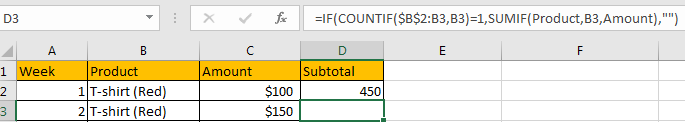In logical test part, CONTIF(\$B\$2:B3,B3) is equal to COUNTIF({“T-shirt (Red)”;”T-shirt (Red)”},”T-shirt (Red)”).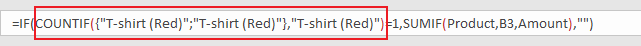Obviously, this formula returns 2, which is not equal to 1, so IF function returns ‘valus_if_false’ value, which is “” an empty value in this case. Above all, in D3, the subtotal is blank.

## SUMMARY

1. To subtotal values we can apply different functions together. In this case we apply IF function core formula, and a formula contains COUNTIF function as logical test, SUMIFS function as ‘True’ part returned value.

2. For above three functions, they all allow logical operators like “>”,”>”,”<>” and wildcards like “*”,”?”.

### Related Functions

• Excel IF function
The Excel IF function perform a logical test to return one value if the condition is TRUE and return another value if the condition is FALSE. The IF function is a build-in function in Microsoft Excel and it is categorized as a Logical Function.The syntax of the IF function is as below:= IF (condition, [true_value], [false_value])….
• Excel COUNTIF function
The Excel COUNTIF function will count the number of cells in a range that meet a given criteria. This function can be used to count the different kinds of cells with number, date, text values, blank, non-blanks, or containing specific characters.etc.= COUNTIF (range, criteria)…
• Excel SUMIF Function
The Excel SUMIF function sum the numbers in the range of cells that meet a single criteria that you specify. The syntax of the SUMIF function is as below:=SUMIF (range, criteria, [sum_range])…
• Excel SUMIFS Function
The Excel SUMIFS function sum the numbers in the range of cells that meet a single or multiple criteria that you specify. The syntax of the SUMIFS function is as below:=SUMIFS (sum_range, criteria_range1, criteria1, [criteria_range2, criteria2], …)…
• Excel SUM function
The Excel SUM function will adds all numbers in a range of cells and returns the sum of these values. You can add individual values, cell references or ranges in excel.The syntax of the SUM function is as below:= SUM(number1,[number2],…)
• Excel SUBTOTAL function
The Excel SUBTOTAL function returns the subtotal of the numbers in a list or database. The syntax of the SUBTOTAL function is as below:= SUBTOTAL (function_num, ref1, [ref2])….

## How to Only Sum Visible Cells/Rows in a Filtered List in Excel

Sometimes we may want to only sum the visible cells in a filtered list or a table. We may notice that if we use SUM function directly with selecting the visible reference in the filtered list, all data in the list (also contains the hidden rows) is included into calculation.

Above all, we need to find a proper function can only sum visible cells in a filtered list and ignore the filtered-out data. Actually, excel built-in SUBTOTAL function can perform calculating like sum data, count, average for both visible & invisible cells or only visible cells properly. As we only want to show you the formula to perform sum visible cells in filter list, so in this article we will only introduce you the basic usage of SUBTOTAL function on sum data. After reading this article, I think you must have a well understanding of SUBTOTAL function, it is flexible for you to use it in your daily work.

EXAMPLE: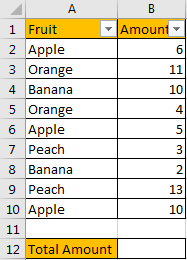If we want to sum total amount for the filtered fruit, can we use SUM function here directly?

Enter =SUM(B2:B10) in B12. See screenshot below, we get 64 here, the returned value is incorrect obviously. It equals to the total amount of the whole list B2:B10, the hidden rows are also taken into calculation.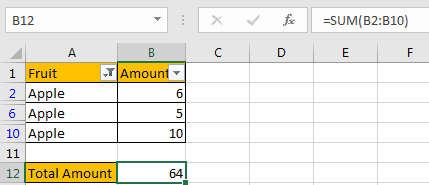FORMULA:

Let’s apply SUBTOTAL function in this case to try to calculate total amount for ‘Apple’ again.

Step 1: Enter =SUBTOTAL(9,B2:B10).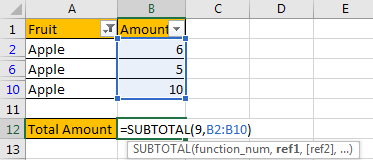Step 2: Press Enter to load return value.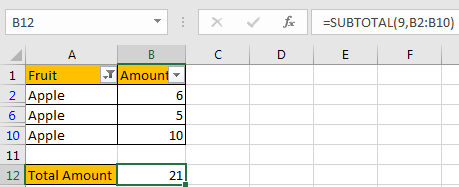We can see that this time correct amount 21 is displayed in B12.

HOW THIS FORMULA WORKS:

This formula only contains only one function: SUBTOTAL function.

Syntax:

=SUBTOTAL (function_num, ref1, [ref2], …)

It has several arguments like function_num, ref1, ref2. For function_num, it is a number, it shows the function used by SUBTOTAL, by providing different numbers, SUBTOTAL function can perform different calculation. As we mentioned above, SUBTOTAL function can perform most basic calculations like SUM, COUNT, AVERAGE, etc., so we need to know some useful function numbers before explaining SUBTOTAL function.

Please see below screenshots, they show the correspondence between number and function.

Function number from 1 – 101.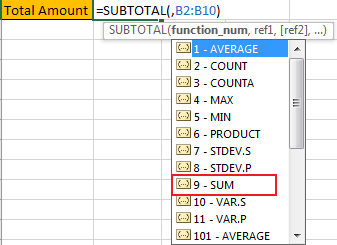Function number from 101 – 111.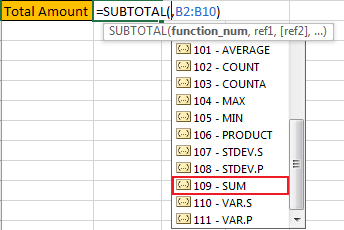Refer to above function number list, we can see that SUM function has two function numbers, 9-SUM and 109-SUM. Actually, if we enter 9, SUBTOTAL function will only sum visible data and ignore the filtered-out data; and if we enter 109, SUBTOTAL function will only sum visible data and ignore the manually hidden data. See, the difference is:

9: ignore data which is hidden by filter list;

109: ignore data which is hidden manually.

In this instance, in the formula =SUBTOTAL(9,B2:B10). There are two arguments. For the first argument function_num, as data is hidden by filter list, so we enter 9 here; for the second argument, reference, we can enter the whole list B2:B10, or you can also hold your mouse and select the visible cells directly.

RESULT:

We already get proper amount for ‘Apple’, this time let’s pick Banana to check if our formula works properly after changing the filter criteria.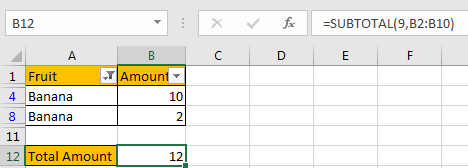It works properly. SUBTOTAL function can work well on ‘sum visible cells’.

NOTES:

1. If we change function_num from 9 to others, the SUBTOTAL function will change calculation accordingly based on the function number. For example, change 9 to 2 in this case (2 – COUNT).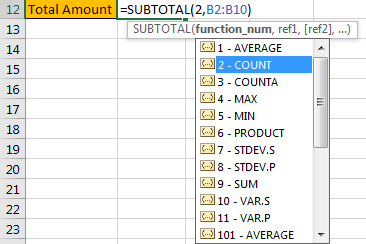We will get 2 by applying the formula.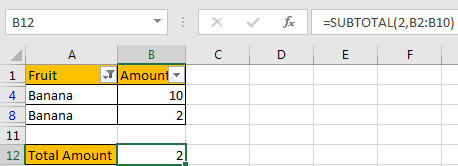1. SUBTOTAL function can ignore all hidden data, so we can also use it to sum visible cells in a range. Just enter the range as the reference, the it will ignore hidden cells in this range.

### Related Functions

• Excel SUBTOTAL function
The Excel SUBTOTAL function returns the subtotal of the numbers in a list or database. The syntax of the SUBTOTAL function is as below:= SUBTOTAL (function_num, ref1, [ref2])….
• Excel SUM function
The Excel SUM function will adds all numbers in a range of cells and returns the sum of these values. You can add individual values, cell references or ranges in excel.The syntax of the SUM function is as below:= SUM(number1,[number2],…)…

## How to Sum Only Filtered Data or Visible Cell Values in Excel

This post will guide you how to sum only visible cell values in Excel. How do I sum only visible cell values in a selected range with a formula in Excel. How to sum only visible rows when filtering data in your worksheet with VBA code in Excel.

## Sum Filtered Data Only with Formula

Assuming that you have a list of data in range A1:C6, which contain sales s data. And you have filtered out the data that is greater than 5. Then you need to sum all filtered data only or all visible values without hidden values. How to achieve it. You can use a formula based on the SUBTOTAL function to achieve the result. Like this:

`=SUBTOTAL(9,C2:C6)`

Type this formula into a blank cell and press Enter key to apply this formula. And then all visible value should be summed out.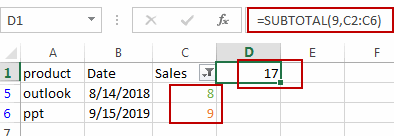## Sum Filtered Data Only with VBA Code

You can also write a User Defined Function with VBA code to achieve the same result. Just do the following steps:

#1 open your excel workbook and then click on “Visual Basic” command under DEVELOPER Tab, or just press “ALT+F11” shortcut.#2 then the “Visual Basic Editor” window will appear.

#3 click “Insert” ->”Module” to create a new module.#4 paste the below VBA code into the code window. Then clicking “Save” button.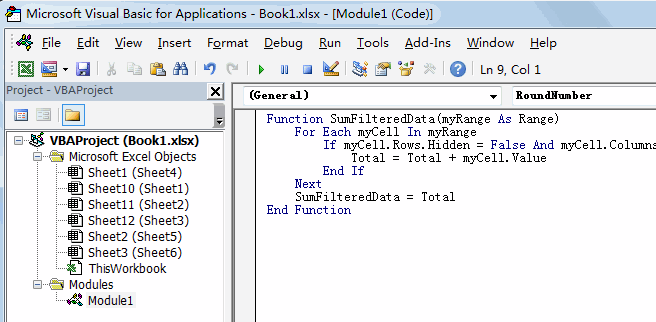```Function SumFilteredData(myRange As Range)
For Each myCell In myRange
If myCell.Rows.Hidden = False And myCell.Columns.Hidden = False Then
Total = Total + myCell.Value
End If
Next
SumFilteredData = Total
End Function```

#5 back to the current worksheet, then type the following formula in a blank cell. press Enter key.

=SumFilteredData(C2:C6)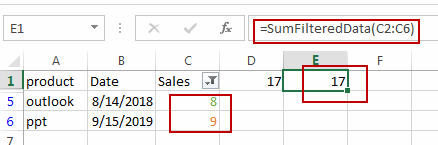Video: Sum Filtered Data Only (Sum Visible Cell values Only)

### Related Functions

• Excel SUBTOTAL function
The Excel SUBTOTAL function returns the subtotal of the numbers in a list or database. The syntax of the SUBTOTAL function is as below:= SUBTOTAL (function_num, ref1, [ref2])….

## How to Count And Sum Cells by Color in Excel

This post will guide you how to count and sum cells by font color or cell color in Excel. How do I sum cell values by font color with VBA code in Excel. How to sum cells by color with formula in Excel.

## Sum Cells by Color with Filter feature

Assuming that you have a list of data in range A1:C6, which contain product name and its sale values, and the cells that contain sale values have been colored by different color. And you want to sum sale values by color in your worksheet. How to achieve it. You can use the filter command in combination with SUBTOTAL function to achieve the result. Just do the following steps:

#1 select the sale column in your range. And go to DATA tab, click Filter command under Sort & Filter group. And one filter icon will be added in the first cell of sales column.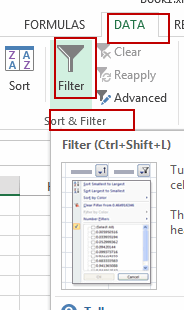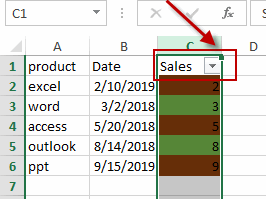#2 click filter icon on the Cell C1, and click Filter by Color, then select one color that you want to be filtered (such as: select green color as filtered color). Click Ok button.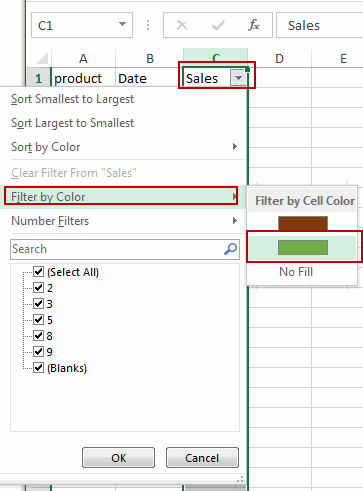#3 all cell will be filtered out by green color.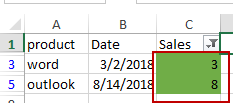#4 Type the following formula in a blank cell to sum cells by green color, and then press Enter key in your keyboard.

`=SUBTOTAL(9,A2:C6)`

You will see that cell values will be counted by green color only.

##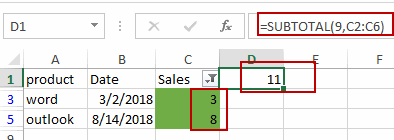Sum Cells by Color with VBA Code

You can also write a User Defined Function to achieve the result of summing cells by Cell color in Excel. Just do the following steps:

#1 open your excel workbook and then click on “Visual Basic” command under DEVELOPER Tab, or just press “ALT+F11” shortcut.#2 then the “Visual Basic Editor” window will appear.

#3 click “Insert” ->”Module” to create a new module.#4 paste the below VBA code into the code window. Then clicking “Save” button.

##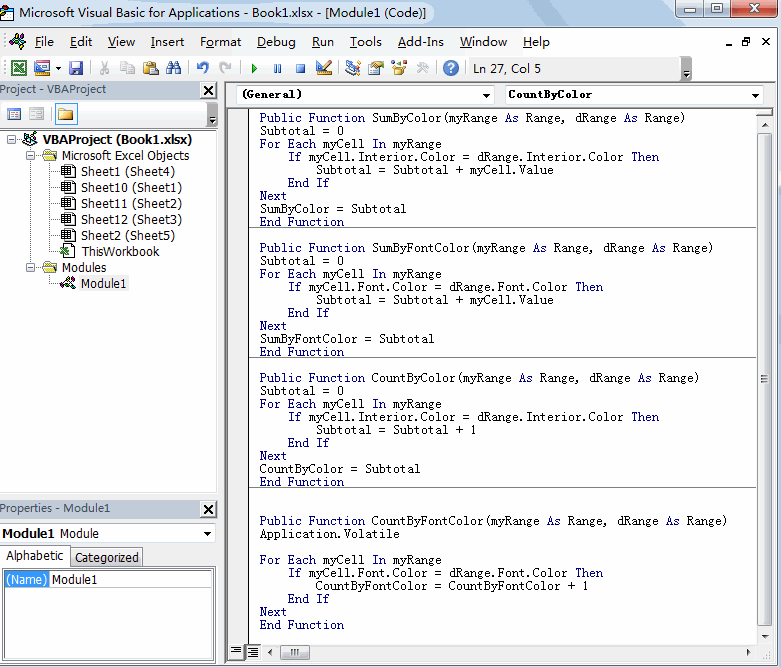```Public Function SumByColor(myRange As Range, dRange As Range)
Subtotal = 0
For Each myCell In myRange
If myCell.Interior.Color = dRange.Interior.Color Then
Subtotal = Subtotal + myCell.Value
End If
Next
SumByColor = Subtotal
End Function```

#5 back to the current worksheet, then type the following formula in a blank cell. press Enter key.

`=SumByColor(C2:C6,C3)`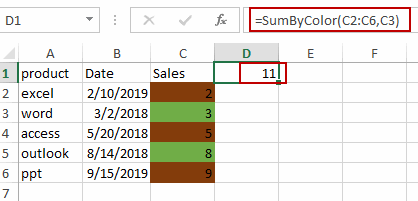## Sum Cells by Font Color with VBA Code

If you want to sum cells by Font color for in a given range of cells in Excel, you can also write a User Defined function to achieve the result. Just do the following steps:

#1 repeat the above steps 1-3.

#2 paste the below VBA code into the code window. Then clicking “Save” button.

```Public Function SumByFontColor(myRange As Range, dRange As Range)
Subtotal = 0
For Each myCell In myRange
If myCell.Font.Color = dRange.Font.Color Then
Subtotal = Subtotal + myCell.Value
End If
Next
SumByFontColor = Subtotal
End Function```

#3 back to the current worksheet, then type the following formula in a blank cell. press Enter key.

=SumByFontColor(C2:C6,C3)

## Count Cells by Color with VBA Code

If you only want to count cells by one color in your range of cells, you can use a User Defined Function to achieve the result. Just do the following steps:

#1 open your excel workbook and then click on “Visual Basic” command under DEVELOPER Tab, or just press “ALT+F11” shortcut.#2 then the “Visual Basic Editor” window will appear.

#3 click “Insert” ->”Module” to create a new module.#4 paste the below VBA code into the code window. Then clicking “Save” button.

```Public Function CountByColor(myRange As Range, dRange As Range)
Subtotal = 0
For Each myCell In myRange
If myCell.Interior.Color = dRange.Interior.Color Then
Subtotal = Subtotal + 1
End If
Next
CountByColor = Subtotal
End Function```

#5  back to the current worksheet, then type the following formula in a blank cell. press Enter key.

=CountByColor(C2:C6,C3)

## Count Cells by Font Color with VBA Code

If you want to count cells by Font Color, you can use the below User Defined function to achieve the result.

```Public Function CountByFontColor(myRange As Range, dRange As Range)
Application.Volatile

For Each myCell In myRange
If myCell.Font.Color = dRange.Font.Color Then
CountByFontColor = CountByFontColor + 1
End If
Next
End Function```

##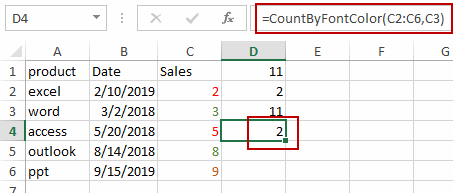Video: Count and Sum Cells by Color

### Related Functions

• Excel SUBTOTAL function
The Excel SUBTOTAL function returns the subtotal of the numbers in a list or database. The syntax of the SUBTOTAL function is as below:= SUBTOTAL (function_num, ref1, [ref2])….

## Count Blank or Non-blank Cells in Filtered Range

This post will guide you how to count blank or empty cells in a filtered range of cells in excel. How do I count non-blank cells in a filtered range of cells in excel. How to count blank or non-blank cells in a filtered list with SUBTOTAL function in a worksheet.

## Count Blank Cells

If you want to count only the visible blank cells in a filtered list in your worksheet, you can use a formula based on the SUBTOTAL function. For example, you have a filtered range of cells B1:C7, and those two columns have been filtered.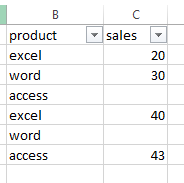And you want to get the number of all visible blank cells in column C. you just need to use the following formula:

`=SUBTOTAL(3,B2:B7)-SUBTOTAL(3,C2:C7)`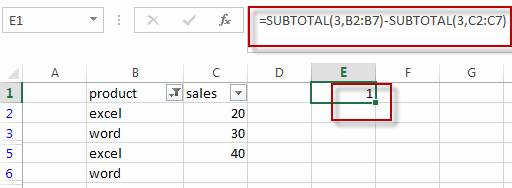Type this formula into a blank cell and then press Enter key in your keyboard. You will see the number of all visible blank cells in your current filtered cells.

## Count non-blank Cells

If you want to count the number of all visible non-blank cells in a filtered range, you can use the following formula based on the SUBTOTAL function.

`=SUBTOTAL(102,C2:C5)`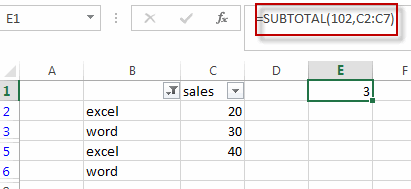### Related Functions

• Excel SUBTOTAL function
he Excel SUBTOTAL function returns the subtotal of the numbers in a list or database. The syntax of the SUBTOTAL function is as below:= SUBTOTAL (function_num, ref1, [ref2])….

## How To Use Excel SUBTOTAL Function

This post will guide you how to use Excel SUBTOTAL function with syntax and examples in Microsoft excel.

## Description

The Excel SUBTOTAL function returns the subtotal of the numbers in a list or database.

The SUBTOTAL function is a build-in function in Microsoft Excel and it is categorized as a Math and Trigonometry Function.

The SUBTOTAL function is available in Excel 2016, Excel 2013, Excel 2010, Excel 2007, Excel 2003, Excel XP, Excel 2000, Excel 2011 for Mac.

## Syntax

The syntax of the SUBTOTAL function is as below:

`= SUBTOTAL (function_num, ref1, [ref2])`

Where the SUBTOTAL function argument is:
Function_num – This is a required argument.  It can be set as 1-11 or 101-111 for the subtotal.  1-11 that includes hidden rows and 101-111 excludes hidden rows.

 Function_num (includes hidden values) Function_num (ignores hidden values) Function 1 101 AVERAGE 2 102 COUNT 3 103 COUNTA 4 104 MAX 5 105 MIN 6 106 PRODUCT 7 107 STDEV 8 108 STDEVP 9 109 SUM 10 110 VAR 11 111 VARP

Ref1– This is a required argument. The first named range or reference that you want to subtotal.

## Example

the below examples will show you how to use Excel SUBTOTAL function to return the subtotal of the numbers in a list.

#1 =SUBTOTAL(103,B:B)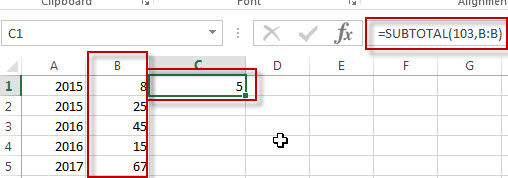Note: the above formula will call COUNTA function to count the number of cells(B:B) that contain numbers. It will return value 5.

#2 =SUBTOTAL(109,B:B)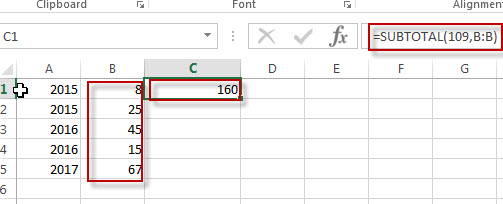Note: the above excel formula will call SUM function to add all numbers in range cell B:B, so it will return value: 160.